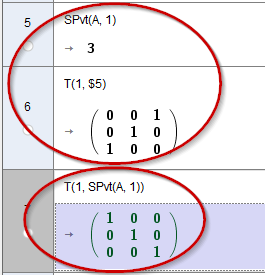# CAS userfunction cal userfunction

hawe shared this problem 1 year ago
Not a Problem

Line 7 userfunction SPvt(A,1) embedded in userfunction T(1, SPvt(A, 1)) does not return correct value (Line 5,6)Line 9 userfunction SPvt(A,1) packed in an if() clause return correct1

That syntax doesn't work in the CAS View, it's Algebra View only. You can check with

`If(false,1,false,2,true,pi,pi/2)`1

If-Clause return correct matrix T(1,3) exchange row 1<->3! (see line 6 and 9)

>{{0, 0, 1}, {0, 1, 0}, {1, 0, 0}}

the nested userfunction call does not work!

SPvt(A, 1) = 3 should be the correkt return value (see line 5), but

T(1, SPvt(A, 1))

> {{1, 0, 0}, {0, 1, 0}, {0, 0, 1}}

Identity matrix is wrong - should be matrix T(1,3) exchange row 1<->3 seems as return of SPvt(A, 1) = 1

If you change

A:={{4,6,-2},{7,3,8},{-6,-1,5}}

(5) SPvt(A, 1)=2

then Lines 6 and 8 responding and return a matrix exchange 1<->2

but line 7 (T(1, SPvt(A, 1))) does not respond and return still identity matrix